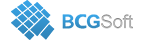BCGSuite for MFC
 void CBCGPChartTransitionFormula::SetTransitionType ( TransitionType type, LPARAM lParam = `NULL`, BOOL bGenerateDataPoints = `TRUE` )

Sets a new predefined transition type.

Call this method to set a new predefined transition type.

If a formula has already been set to a series with CBCGPChartSeries::SetFormula, you need to obtain a pointer to formula object using CBCGPChartSeries::GetFormula.

Copy
CBCGPChartTransitionFormula* pFormula = DYNAMIC_DOWNCAST(CBCGPChartTransitionFormula, pSeriesResult->GetFormula());
// clear a callback if it was set previously
pFormula->SetTransitionCallback(NULL);

type can be one of the following enumerated values:

• TT_SUM - formula will calculate sum of similar (located at the same index) data points for all input series.
• TT_DIFF - difference
• TT_HIGH - high value (max)
• TT_LOW - low value (min)
• TT_MULTIPLY - multiply
• TT_DIVIDE - divide
• TT_AVERAGE - average
Parameters
 type Specifies the transition type. lParam Specifies user-defined data. bGenerateDataPoints TRUE - recalculate output data points immediately.